Courses

# Dimensional And Model Analysis - 1

## 10 Questions MCQ Test Mock Test Series for Civil Engineering (CE) GATE 2020 | Dimensional And Model Analysis - 1

Description
This mock test of Dimensional And Model Analysis - 1 for Civil Engineering (CE) helps you for every Civil Engineering (CE) entrance exam. This contains 10 Multiple Choice Questions for Civil Engineering (CE) Dimensional And Model Analysis - 1 (mcq) to study with solutions a complete question bank. The solved questions answers in this Dimensional And Model Analysis - 1 quiz give you a good mix of easy questions and tough questions. Civil Engineering (CE) students definitely take this Dimensional And Model Analysis - 1 exercise for a better result in the exam. You can find other Dimensional And Model Analysis - 1 extra questions, long questions & short questions for Civil Engineering (CE) on EduRev as well by searching above.
QUESTION: 1

Solution:
QUESTION: 2

### A spillway of an irrigation project is to be studied by means of a model constructed to a scale of 1 : 9. The prototype discharge is 1000 m3/s. Neglecting the viscous and surface tension effects, the required flow rate for the model is

Solution: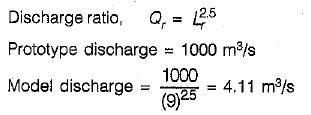QUESTION: 3

### Match List-I (Equation/Principie) with List-ll (Property) and select the correct answer using. the codes given below the lists: List-I A. Energy equation B. Continuity equation C. Moment of momentum principle D: Impulse-momentum principle List-ll 1. Force on a moving vane 2. Lawn sprinkler 3. Pressure at a point in a pipeline 4. Flow at two sections of a tapering pipe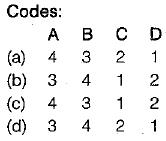Solution:
QUESTION: 4

The distorted models, used in the hydraulic studies, are those which

Solution:

Distorted - model can have vertical scale exaggeration or slope exaggeration. Therefore they are not geometrically similar. It may have either geometrical distortion, or material distortion or distortion of hydraulic quantities or a combination of these.

QUESTION: 5

A 1: 30 scale model of a submarine is to be tested in a wind tunnel for its drag when it is operating at 15 km/h in ocean. The kinematic viscosity of air is 1.51 x 10-5 m2/s and for water 1.02 x 10-6 m2/s. What is the velocity of air in wind tunnel that should be maintained for kinematic similarity?

Solution:

The Reynold’s model law shall be applied in the case of fully submerged objects,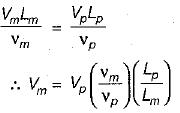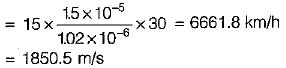The Reynold’s model law ensures dynamic; kinematic and geometric similarities.

QUESTION: 6

In model similarity, if gravitational and inertial forces are the only important forces, then what is the discharge ratio?
where Lr = ratio of length dimension.

Solution:

If gravitational and inertial forces are the only important forces, then the Froude number must be the same in the model and prototype. Thus,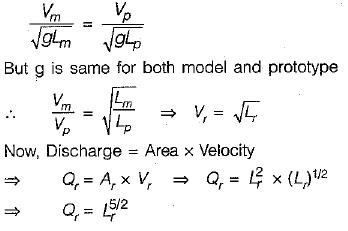QUESTION: 7

Which ratio is the Reynold's Number?

Solution: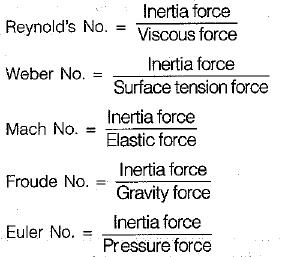QUESTION: 8

An orifice is discharging under a head of 1.25 m of water. A Pitot tube kept at its centre line at the vena contracta indicates a heap of 1.20 m of water. The coefficient of velocity of the orifice is

Solution:

h0 be the head of Orifice
hp be the head of Pitot tube
Velocity of emergence of water from orifice can be given by  =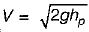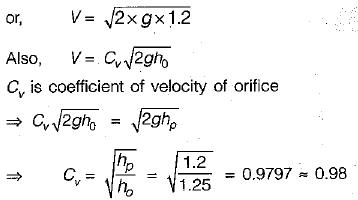QUESTION: 9

The geometrical similarity between model and prototype is achieved by

Solution:

Geometric similarity exists between the model and the prototype if the ratios of corresponding length dimensions in the model and the prototype are equal. Different model scales for horizontal and vertical dimension are used in a distorted model. Geometric similarity is achieved by using same model scale throughout.

QUESTION: 10

Froude number is the ratio of inertia force to

Solution: